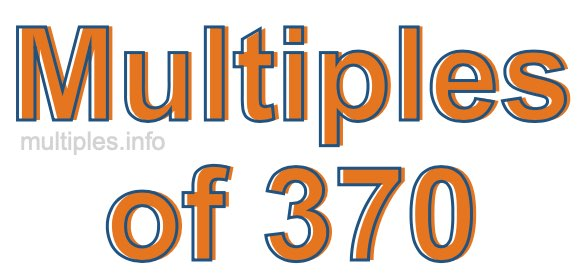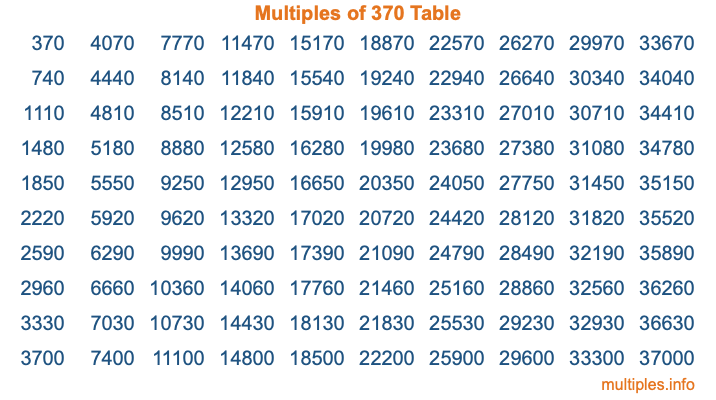Multiples of 370Welcome to the Multiples of 370 page. Here we will first teach you everything you will ever need to know about the multiples of 370, and then give you a study guide summary of everything we taught you to make sure you remember it all. Use this page to look up facts and learn information about the multiples of 370. This page will make you a multiples of three hundred seventy expert!

Definition of Multiples of 370
Multiples of 370 are all the numbers that when divided by 370 equal an integer. Each of the multiples of 370 are called a multiple. A multiple of 370 is created by multiplying 370 by an integer.

Therefore, to create a list of multiples of 370, you start with 1 multiplied by 370, then 2 multiplied by 370, then 3 multiplied by 370, and so on for as long as you want. Thus, the list of the first five multiples of 370 is 370, 740, 1110, 1480, and 1850. To see a larger list of multiples of 370, see the printable image of Multiples of 370 further down on this page. We also have a category where you can choose any nth multiple of 370.

Multiples of 370 Checker
The Multiples of 370 Checker below checks to see if any number of your choice is a multiple of 370. In other words, it checks to see if there is any number (integer) that when multiplied by 370 will equal your number. To do that, we divide your number by 370. If the the quotient is an integer, then your number is a multiple of 370.

Is  a multiple of 370?

Least Common Multiple of 370 and ...
A Least Common Multiple (LCM) is the lowest multiple that two or more numbers have in common. This is also called the smallest common multiple or lowest common multiple and is useful to know when you are adding our subtracting fractions. Enter one or more numbers below (370 is already entered) to find the LCM.

Check out our LCM Calculator if you need more details about the Least Common Multiple or if you need the LCM for different numbers for adding and subtraction fractions.

nth Multiple of 370
As we stated above, 370 is the first multiple of 370, 740 is the second multiple of 370, 1110 is the third multiple of 370, and so on. Enter a number below to find the nth multiple of 370.

th multiple of 370

Multiples of 370 vs Factors of 370
370 is a multiple of 370 and a factor of 370, but that is where the similarities end. All postive multiples of 370 are 370 or greater than 370. All positive factors of 370 are 370 or less than 370.

Below is the beginning list of multiples of 370 and the factors of 370 so you can compare:

Multiples of 370: 370, 740, 1110, 1480, 1850, etc.

Factors of 370: 1, 2, 5, 10, 37, 74, 185, 370

As you can see, the multiples of 370 are all the numbers that you can divide by 370 to get a whole number. The factors of 370, on the other hand, are all the whole numbers that you can multiply by another whole number to get 370.

It's also interesting to note that if a number (x) is a factor of 370, then 370 will also be a multiple of that number (x).

Multiples of 370 vs Divisors of 370
The divisors of 370 are all the integers that 370 can be divided by evenly. Below is a list of the divisors of 370.

Divisors of 370: 1, 2, 5, 10, 37, 74, 185, 370

The interesting thing to note here is that if you take any multiple of 370 and divide it by a divisor of 370, you will see that the quotient is an integer.

Multiples of 370 Table
Below is an image of the first 100 multiples of 370 in a table. The table is in chronological order, column by column. The first column has the first ten multiples of 370, the second column has the next ten multiples of 370, and so on.The Multiples of 370 Table is also referred to as the 370 Times Table or Times Table of 370. You are welcome to print out our table for your studies.

Negative Multiples of 370
Although not often discussed or needed in math, it is worth mentioning that you can make a list of negative multiples of 370 by multiplying 370 by -1, then by -2, then by -3, and so on, to get the following list of negative multiples of 370:

-370, -740, -1110, -1480, -1850, etc.

Multiples of 370 Summary
Below is a summary of important Multiples of 370 facts that we have discussed on this page. To retain the knowledge on this page, we recommend that you read through the summary and explain to yourself or a study partner why they hold true.

There are an infinite number of multiples of 370.

A multiple of 370 divided by 370 will equal a whole number.

370 divided by a factor of 370 equals a divisor of 370.

The nth multiple of 370 is n times 370.

The largest factor of 370 is equal to the first positive multiple of 370.

370 is a multiple of every factor of 370.

370 is a multiple of 370.

A multiple of 370 divided by a divisor of 370 equals an integer.

370 divided by a divisor of 370 equals a factor of 370.

Any integer times 370 will equal a multiple of 370.

Multiples of a Number
Here you can get the multiples of another number, all with the same attention to detail as we did for multiples of 370 on this page.

Multiples of
Multiples of 371
Did you find our page about multiples of three hundred seventy educational? Do you want more knowledge? Check out the multiples of the next number on our list!